﻿ Appendix (QED)

# Appendix of QED g-2 factor calculation

## Conversion of Notations from ( -1, +1, +1, +1 ) to ( +1, -1, -1, -1 ) versions.

On this web site, we use ( -1, +1, +1, +1) or (-+++) version of metric tensor.
So only the sign of the zero component of the four-vector changes by the index positions.
If you want to change to ( +1, -1, -1, -1 ) or (+---) version of metric tensor or signature, do as shown in Eq.1, Eq.2 and Fig.1-.

(Eq.1)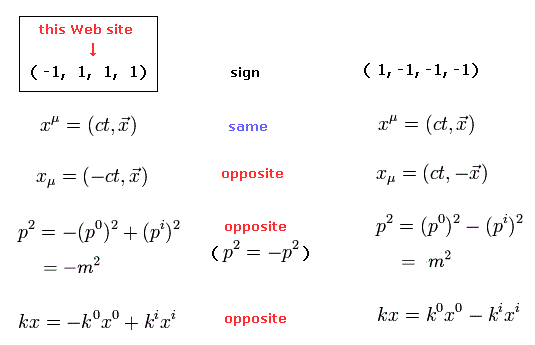( xμ, pμ, and kμ = four-vectors )

And the matrices are
(Eq.2)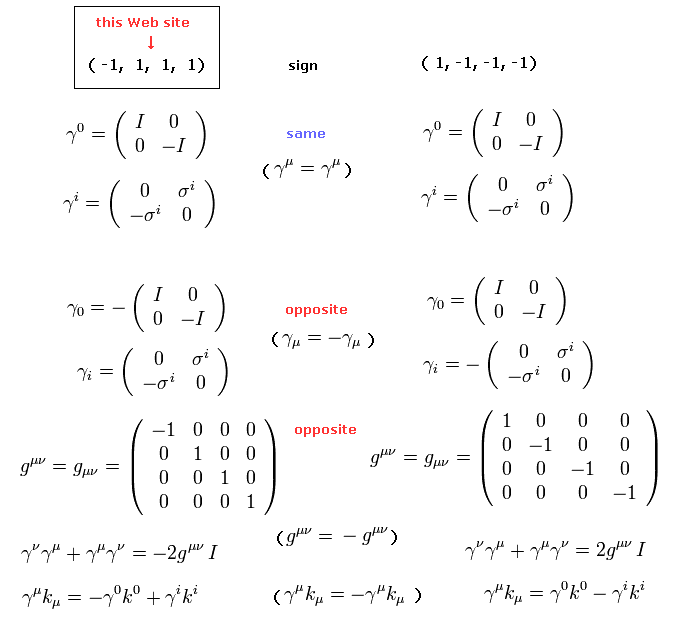Basically, the meaning of the equations is completely the same in both ( -1, +1, +1, +1 ) and ( +1, -1, -1, -1 ) versions.
Only the notations are different in them.
So change only the signs ( + → -, or - → +) when encountering the letters with subindex like pμ → -pμ, and  p2 (= pμpμ ) → -p2.

## Notation of relativistic four vectors

(Fig.1) time (= t or x0 ) and space (= xj or x,y,z ) variablesThis website uses the metric signatrue of (-1,1,1,1) or (-+++) where only the sign of zero component vector (= time ) with subindex changes (= ct = x0 = -x0, where x0 = -ct ), while the space-component vectors do not change ( xj = xj,  j = 1,2,3 ) their signs regardless of whether subindex or superscript in the spacetime four vectors ( this p.3,  this p.3 ).

If you want to switch from (-1,1,1,1) or (-+++) into (1,-1-1-1) or (+---) version of metric signature, change the sign only of the space coordinate or 1-3 components ( xj = -xj  j = 1,2,3 ) instead of the time variable or zero-component of four vectors.

## Relativistic energy (= p0 = E/c ) and momentum (= pj   j = 1,2,3 )

(Fig.2) "c" is light speed.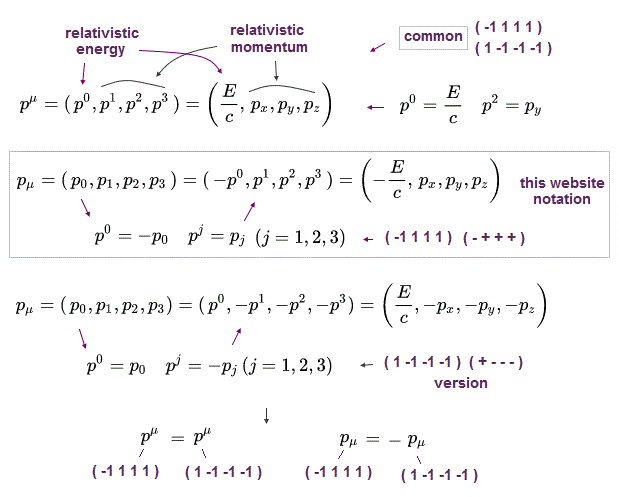Fig.2 is the relativistic energy (= E = cp0 ) and momentum (= p1, p2, p3 or px, py, pz ).

If you want to switch from this website's (-1,+1,+1,+1) or (-+++) version of metric signature into (1,-1,-1,-1) or (+---) version, change only the sign of letters with subscript like pμ → - pμ

## Relativistic mass (= m ), energy (= E ), momentum (= p ) relation.

(Fig.3)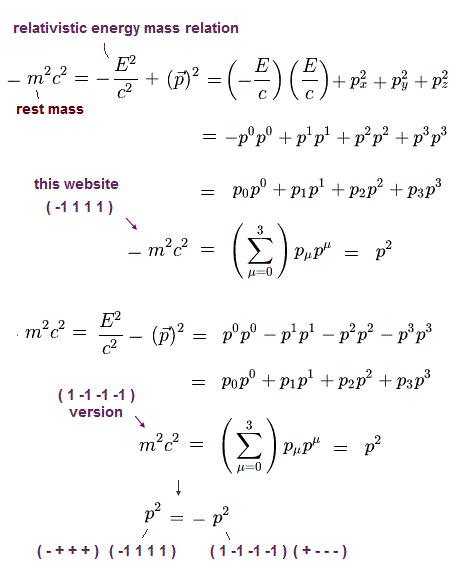Using Fig.2, relativistic energy-momentum-mass relation is expressed like Fig.3.

In this notation rule, when the same letter is used twice in one term like pμpμ or p2, it means the sum of all μ = 0,1,2,3 components (= even when the summation simbol Σ is not added ) like the upper figure ( this p.9,  this p.3-4,  this p.2 ).

## Differential operator notation.

(Fig.4)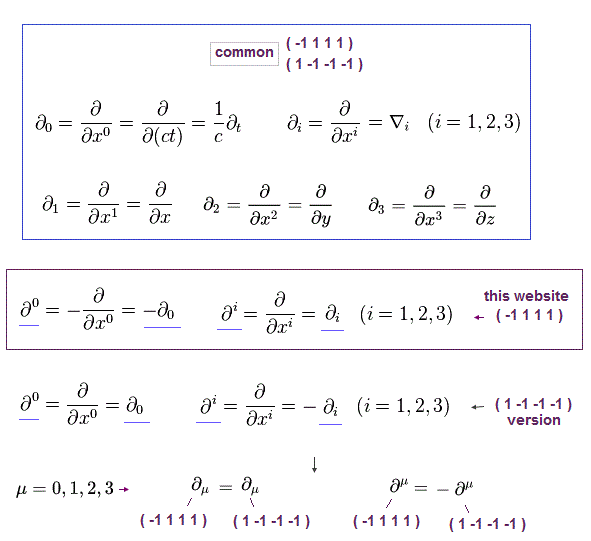In the derivative (= ∇ or ∂ ) or differential operators, when the index is at the lower position (= subindex or subscript ), it means the common normal differential operator.

When the index position is at the higher position (= superscript ), the sign of only the zero component (= time ) differential operator switches into the negative, while the signs of all other space derivatives remain the same irrespective of the index position in this website (-1,1,1,1) version of metric signature.

In the (1,-1-1-1) version of metric signature, the sign of the differential operator with superscript besomes the opposite where only space differential operators become negative, while the sign of the zero-component time differential operator does not change irrespective of the index position ( this p.2 ).

(Fig.5)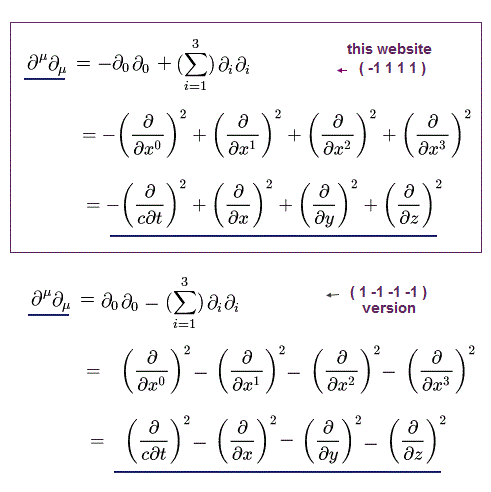## Energy momentum differential representation.

(Fig.6)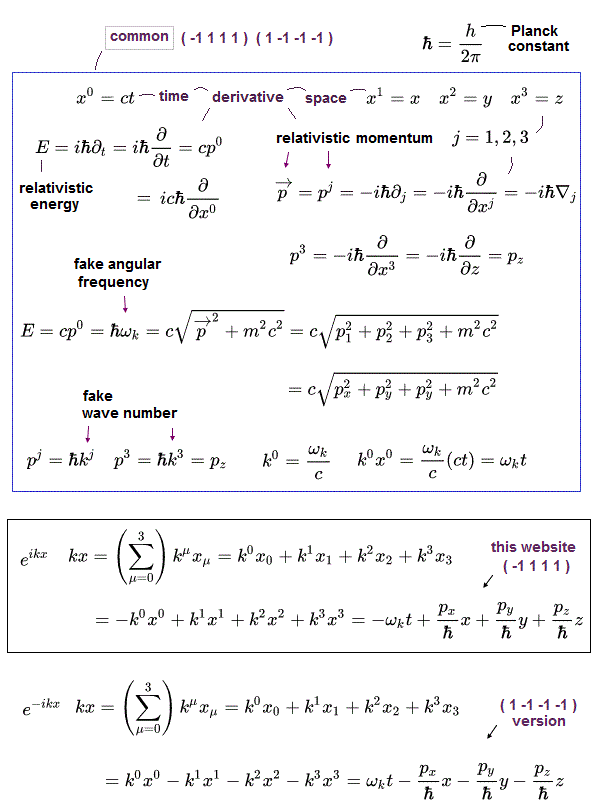Relativistic energy (= E = ℏω where ω is relativistic fictitious angular frequency, ℏ = h/2π where h is Planck constant ) and momentum (= p ) can be expressed as differential operators together with the exponential function of the relativistic wavefunctions ( this p.2 ), based on de Broglie wavelength theory.

## Electric (= φ ) and magnetic (= A ) vector potential and four-currents (= J )

Here we use "four-vector potential (= this vector potential is an invisible fictitious potential introduced only for nonphysical theoretical calculations )" like current density, as follows,
(Fig.7)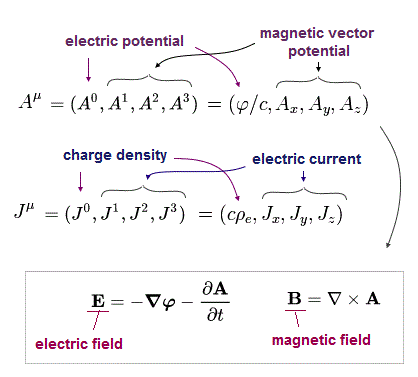In Fig.7, "A" is vector (magnetic) potential allegedly used for expressing the magnetic field B, and "φ" is scalar (electric) potential allegedly used for expressing the electric field E.

(Fig.8) On this website (-1,1,1,1), only the zero-component changes its sign in its subindex.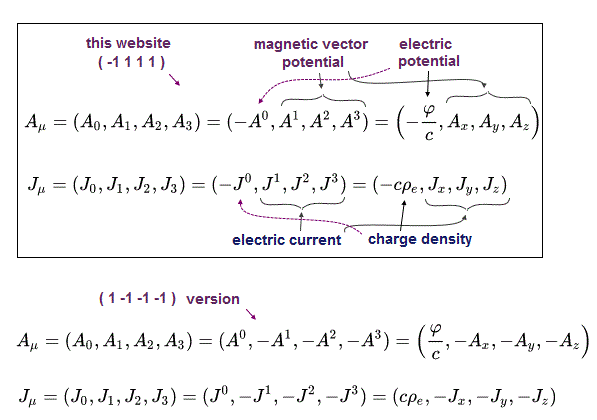## Relativistic Dirac equation's γ matrices.

Relativistic Dirac equation uses nonphysical γ matrices like
(Fig.9)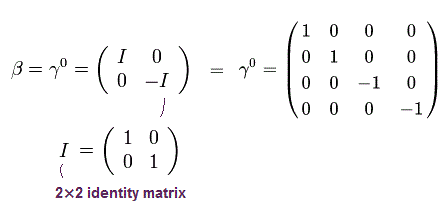where I means 2 × 2 identity matrix.

(Fig.10)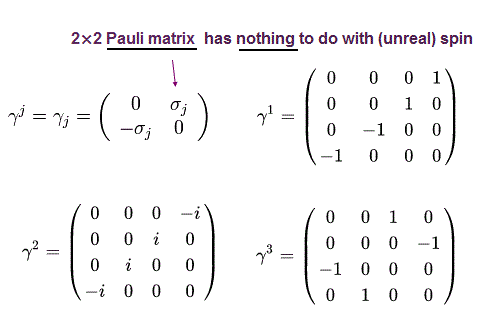where σj mean 2 × 2 Pauli matrices of
(Fig.11)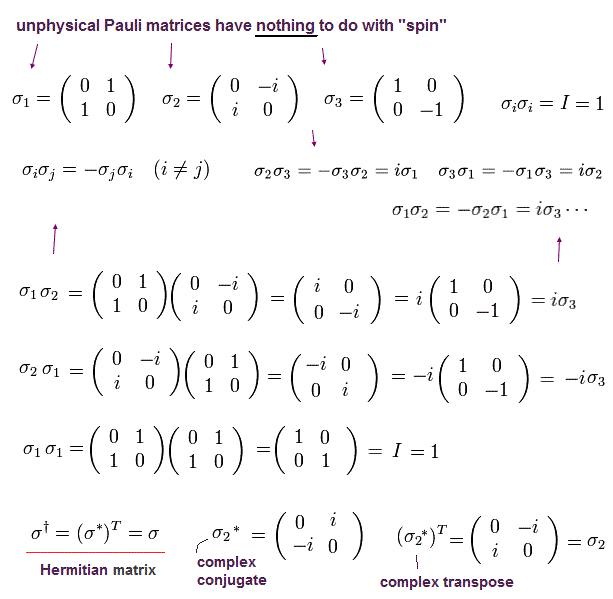γ0γ0 = I ( = 4× 4, identity matrix ),
(Fig.12)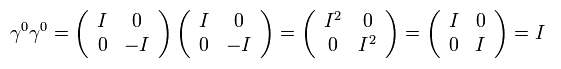And γ matrices satisfy anticommutation relations like,
(Fig.13)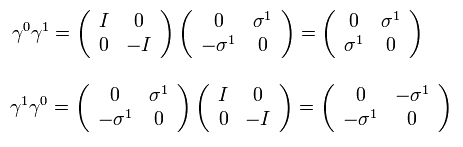(Fig.14)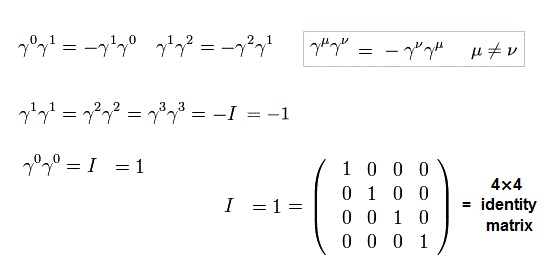(Fig.15)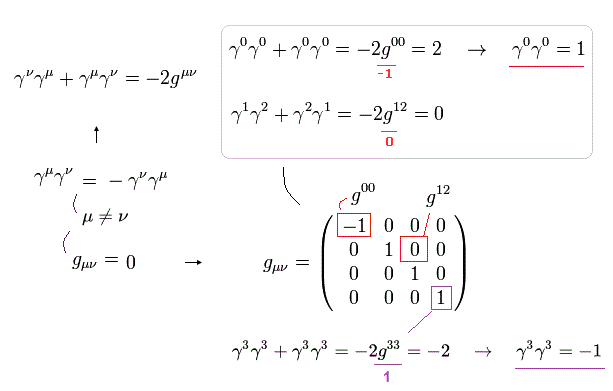## Notation of relativistic Dirac equation.

(Fig.16)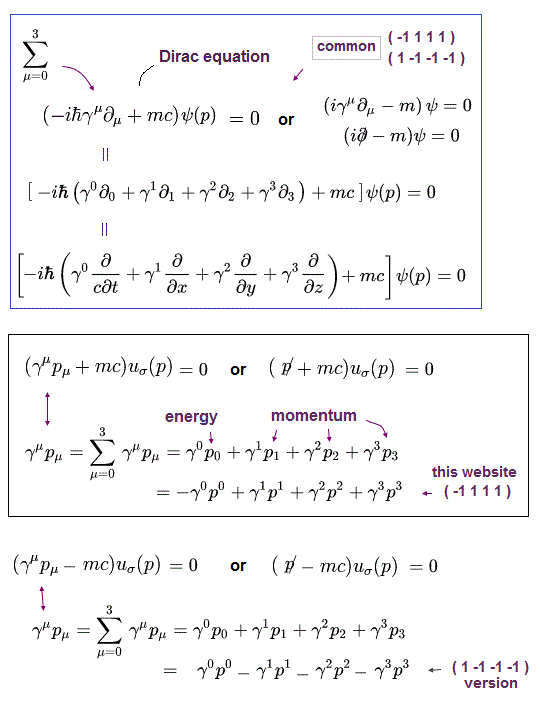Using Fig.2 and Fig.4, the relativistic Dirac equation can be expressed like Fig.16.
(+1,-1,-1,-1) version of Dirac equation is in this p.20,  this p.2-3.

## Relativistic momentum using metric tensor (= gμν ).

(Fig.17)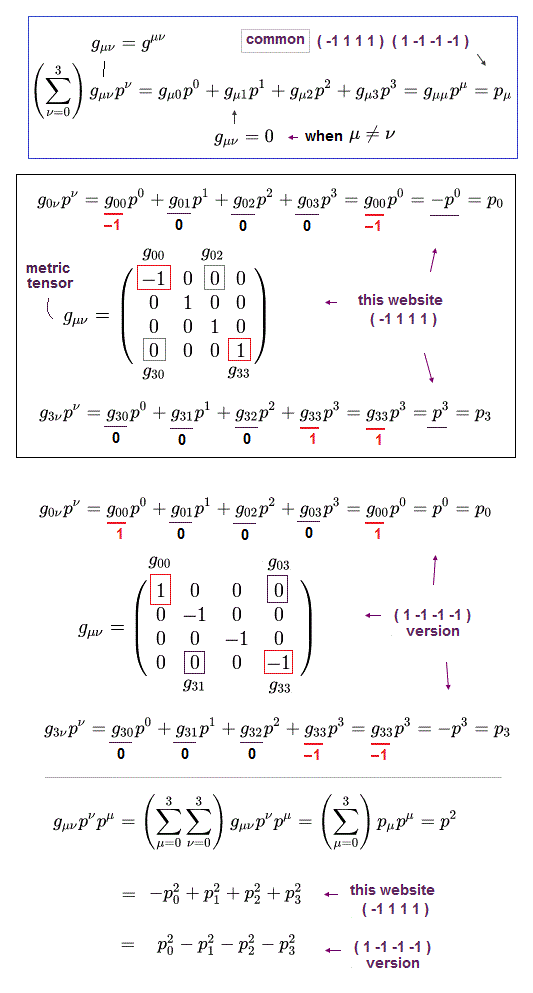Using the metric tensor (= gμν ), the relativistic momentum and energy can be expressed like Fig.17.

## Calculation of Eq.85 of QED g-factor.

Here we prove conversion of the equations in Eq.85 of this page.

(Eq.3) = (Eq.85)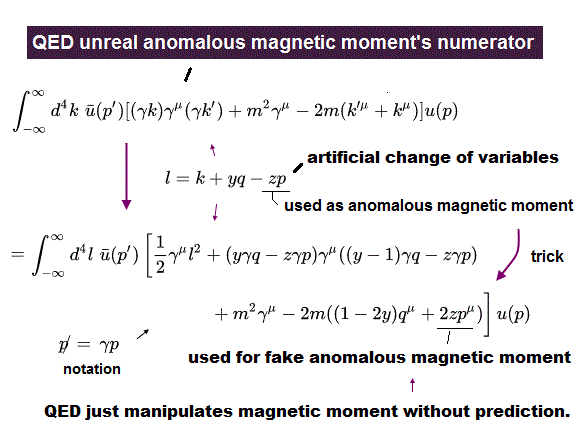(Eq.4)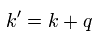In Eq.4, "q" is an external virtual photon, "p" is an incoming electron, and "k" is the internal virtual electron's propagator.
(Eq.5)Using Eq.5, the last terms of Eq.3 ( last equation ) can be expressed as
(Eq.6)So the denominator is a even function of l.

This means that if the numerator is an odd function, integration becomes zero, as follows,
(Eq.7)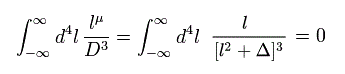Actually, Eq.7 takes the power of infinity.
Remember that the new variable l is made by shifting k by a "finite" value. (See Eq.5.)
If the integral region of k is NOT from -∞ to +∞, this l is neither odd nor even function.
So the convenient tool of Eq.7 depends on infinity.

Using Eq.7, the last term of Eq.6 becomes zero, so the third line of Eq.3 is
(Eq.8)Using Eq.4, the first line of Eq.3 becomes
(Eq.9)As a result, Eq.8 proves to be equal to the last three terms of Eq.9.
As you may notice, this QED method is wrong math, as shown in this.

Using Eq.5, the second term of Eq.3 ( last equation ) becomes
(Eq.10)One term of Eq.10-right-side equation can be expressed as,
(Eq.11)where "a" of left is changed into "b" (= originally, the left and right sides of γμ are independent from each other in the variables "a" of γaka, so the left side "a" is changed into "b" ).
So Eq.11 proves to be equal to the remaining term of Eq.9.

This means the remaing terms of Eq.3 ( last equation ) must be zero.
( So these "zero" terms are added intentionally. )
Other terms of Eq.10 is
(Eq.12)Eq.12 is an odd function of l, so becomes zero by integration.

In the same way, the following term of Eq.10 becomes zero.
(Eq.13)The last term left in Eq.10 is
(Eq.14)Here we use the formula of
(Eq.15)Eq.15 can be proved using the relation of
(Eq.16)Eq.16 means
(Eq.H-1)For example, γ0γ0 = I ( = 4× 4, identity matrix ),
(Eq.H-2)And γ matrices satisfy anticommutation relation like,
(Eq.H-3)Next we prove Eq.15. For exmaple, when μ of Eq.15 is zero,
(Eq.H-4)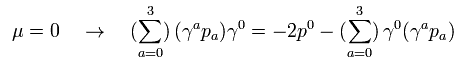Using the relations of Eq.16 and Eq.H-1, the left side of Eq.H-4 is
(Eq.H-5)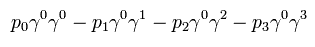where the anticommutation relation is used.

Eq.H-5 is equal to
(Eq.H-6)(Eq.H-7)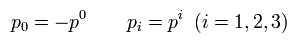As a result, we can prove Eq.H-4.
( In the same way, Eq.15 proves to be true. )

And we also use the formula ( this p.3-(13) ) of
(Eq.17)When μ is different from ν, it means "odd" functions, so Eq.17 becomes zero by integration.

Adding gμν to both sides of Eq.17,
(Eq.17-2)we use
(Eq.17-3)So Eq.17 is right.

Using Eq.15, Eq.14 becomes
(Eq.18)where we change the index "a" of the right γ and k into b (= originally, independent from the left-side a ).

We can change like ( k → l ) of the term which contains one l and one k, as follows,
(Eq.20)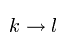Because, the difference between l and k vanishes due to the odd function property of another l.

So the first term of Eq.18 is (using Eq.17 and Fig.17 ),
(Eq.21)where summing 0-3 components with respect to c ( and b).
And only the case of μ = b = c is left, so gμcgbc = 1.

And the second term of Eq.18 becomes
(Eq.22)where γaγb = -gab.
And Eq.17, Eq.16, and Eq.17-3 are used.

Summing the first term of Eq.3 (last equation), Eq.21, and Eq.22,
(Eq.22-2)As a result, we can prove Eq.3.

(Fig.18) Summary of Eq.3 = Eq.10.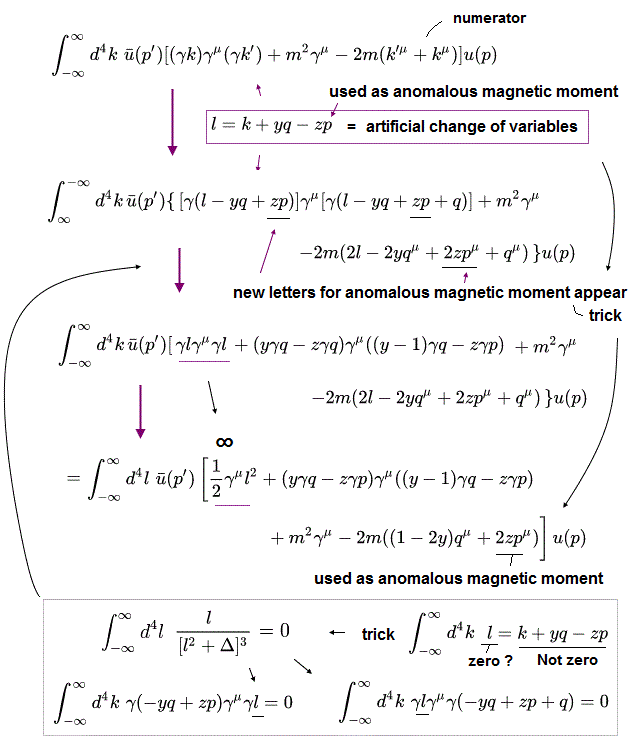## Calculation of Eq.88 of QED g-factor.

Next we try to prove Eq.85 (= Eq.3 ) is equal to Eq.88 (= Eq.23 ) of this page.

(Eq.23) = (Eq.88)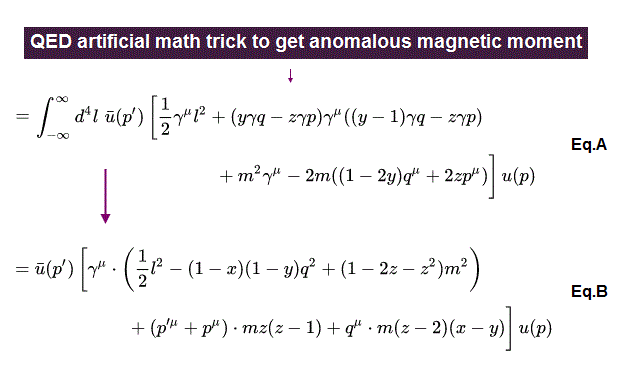Here we need to use the following relations which Dirac equation satisfies. (See this page (Eq.5-12).)
(Eq.5-12)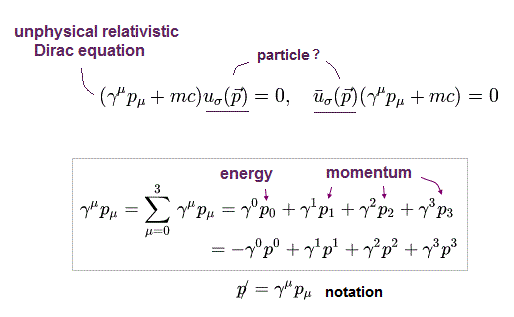(Eq.24)The momentum and energy are conserved, as follows,
(Eq.25)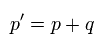In Eq.25, p and p' are "incoming" and "outgoing" electrons, respectively.
The important point is that "q" becomes virtual photon which violates relativity, if Eq.25 is satisfied.

From Eq.24 and E.25,
(Eq.26)Using Eq.24 and Eq.25, the second term of Eq.23 (1) becomes,
(Eq.27)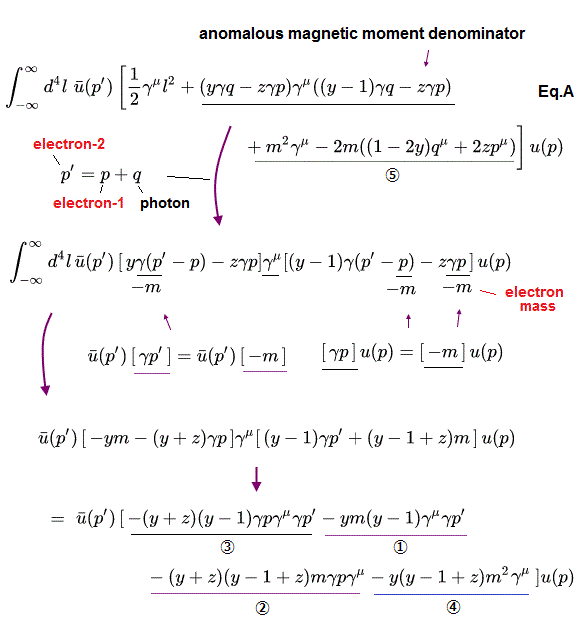(Eq.15)Using Eq.15 and Eq.24, the following term, which is included in the last line of Eq.27, changes into
(Eq.28)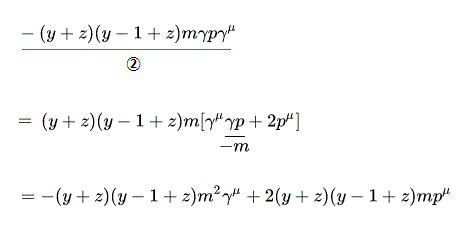And another term, which is included in the last line of Eq.27, becomes
(Eq.29)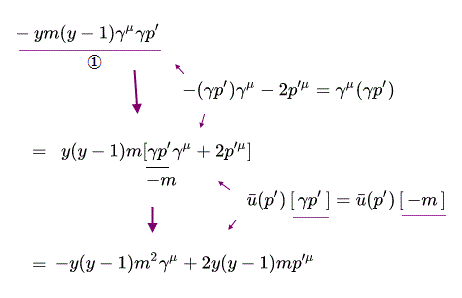As you notice, they try to move p to the right of γμ and p' to the left, and change them into "-m".
So if the virtual photon "q" is zero, p' = p, we don't need to move them, when we try to change them into "-m" (= anomalous magnetic moment value can be freely changed, which is happening in this QED calculation ).

And another term, which is included in the last line of Eq.27 is
(Eq.30)The last one term of Eq.27 is
(Eq.31)Using Eq.15 and Eq.24, one term included in Eq.31 is
(Eq.32)And using Eq.25, another term of Eq.31 is
(Eq.33)where we use the relations of
(Eq.34)And another mass shell condition is
(Eq.35)From Eq.35,
(Eq.36)As I explain later, we do q2 = 0, so, pq = 0, in this case, virtual photon q becomes zero ( q = 0 ).
In this case, g-factor cannot be fixed at a single value.

Summing all calculation values, we can prove Eq.23.
( Using p' = p + q, and x + y + z = 1, and of course, we need to add the remaining terms of Eq.23.)

(Fig.19) Summary of Eq.23Next we sum up the above values to prove Eq.23.
First we calculate the values outside the γμ term (= vertex ).
From the second line of Eq.23-1, we pick up the terms which don't include γ,
(Eq.37)From Eq.28 and Eq.31, pμ temrs are
(Eq.38)From Eq.37 and Eq.38, the sum of pμ temrs are
(Eq.39)From Eq.29 and Eq.32 (= one of Eq.31 ), the sum of p'μ terms are
(Eq.40)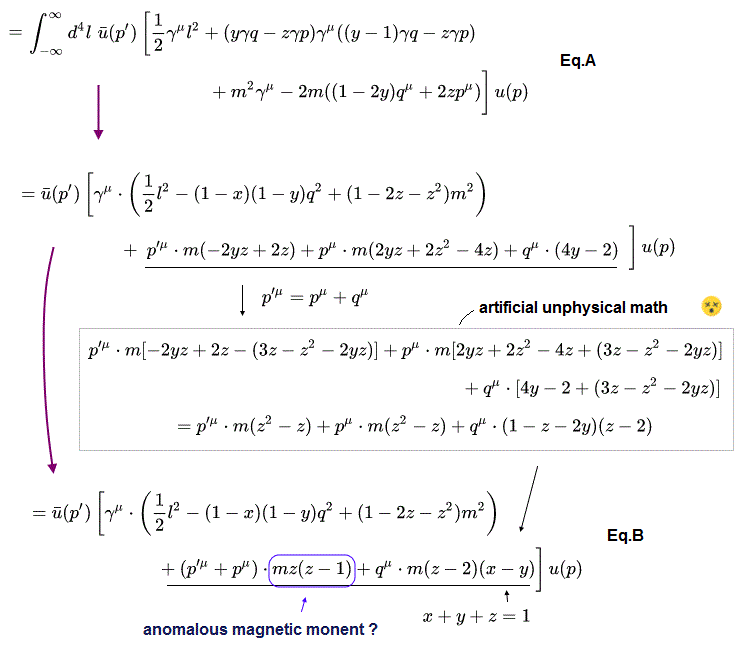where we use p' = p + q.

From Eq.37 and Eq.40, the sum of qμ terms are
(Eq.41)where we use x + y + z = 1.

From Eq.39, Eq.40 and Eq.41, we can get the second line of Eq.23-2.

Next, from Eq.28, Eq.29, Eq.30, Eq.32 (= one of Eq.31), Eq.33 (= one of Eq.31), and the second line of Eq.23-1, the sum of m2 terms in the γ is
(Eq.42)From Eq.33 (= one of Eq.31 ), q2 term in the γ is
(Eq.43)As a result, the first line of Eq.23-2 is obtained.

## Lorentz invariance in propagator.

This section is moved from Eq.4-7 (Eq.4-4) to Eq.4-11 of this page.

(Eq.4-4)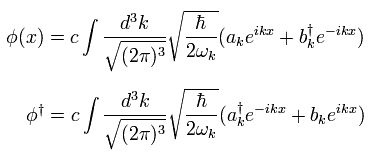For example, when we calculate the next propagator, which means the transmission of the particle,
(Eq.4-7)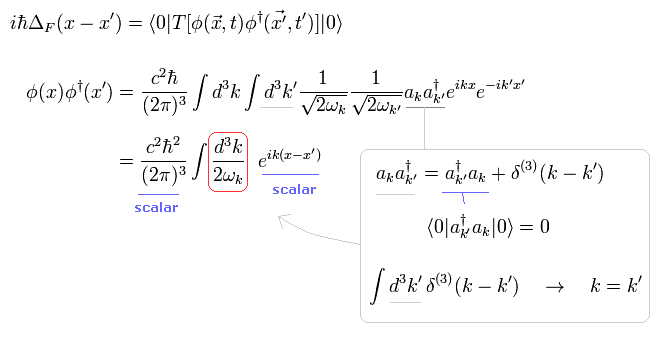where Eq.4-19 is used.
(Eq.4-19)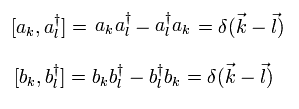Two square roots of ω in Eq.4-4 change to
(Eq.4-8)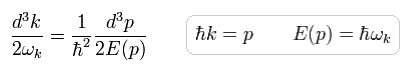After integrating with respect to one d3k , so only another k is left here.

We use the following formula in delta function.
(Eq.4-9)Taking the formula of Eq.4-9, we can get
(Eq.4-10)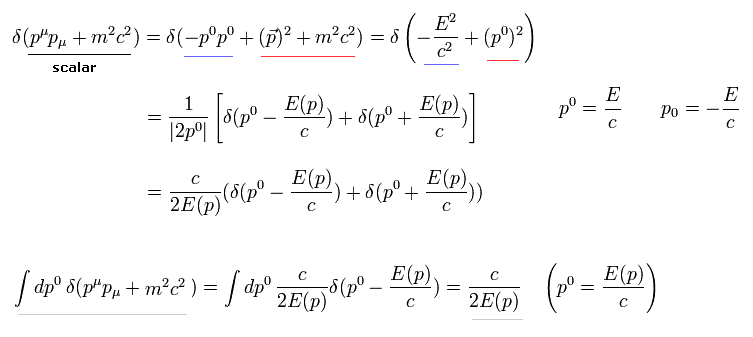where we use Eq.4-1.
(Eq.4-1)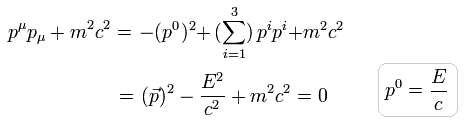Adding d4p to Eq.4-10, and integrating Eq.4-10 with respect to p0, we get the equation of
(Eq.4-11)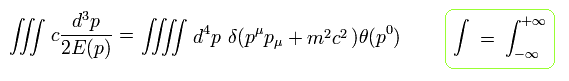where θ means "step function".

Eq.4-11 contanins the integration of d4p and Eq.4-1, so this is Lorentz invariant.
( Of course, integral region must be from minus infinity to plus infinity to make d4p Lorentz invariant. )
So Eq.4-8 is Lorentz-invariant, too.
As you notice, we can change the coefficients such as c and h freely, keeping Lorentz invariance.
So we can adjust these values "artificially" to get the correct energy values.2022/8/27 updated. Feel free to link to this site.